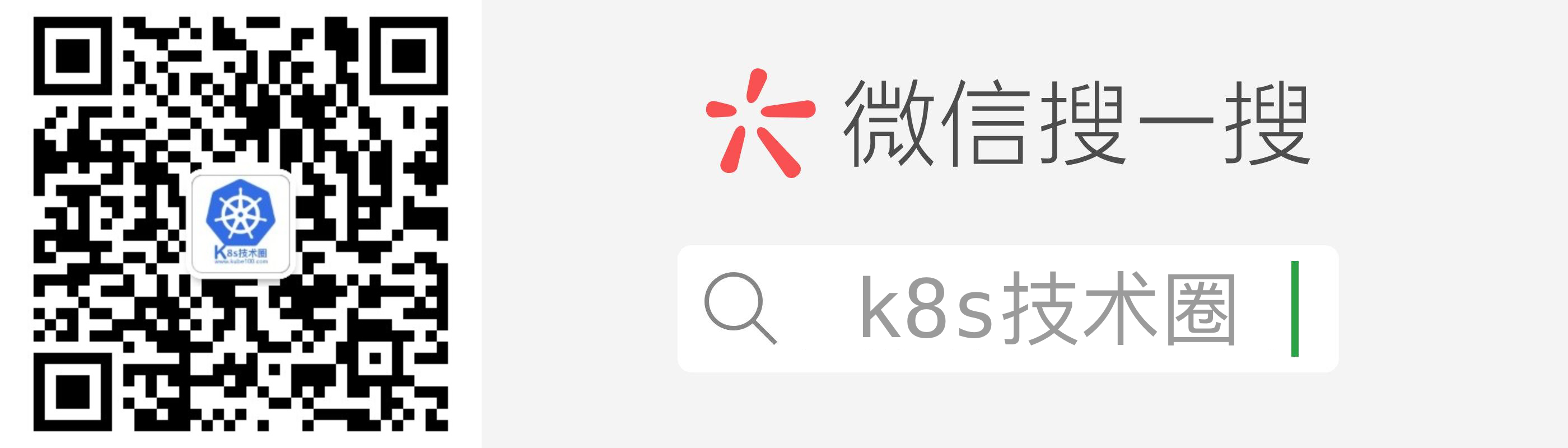# Groovy 简明教程

## 什么是 Groovy

Groovy 是跑在 JVM 中的另外一种语言，我们可以用 Groovy 在 Java 平台上进行编程，使用方式基本与使用 Java 代码的方式相同，所以如果你熟悉 Java 代码的话基本上不用花很多精力就可以掌握 Groovy 了，它的语法与 Java 语言的语法很相似，而且完成同样的功能基本上所需要的 Groovy 代码量会比 Java 的代码量少。

## 环境搭建

``````\$ brew install groovy
``````

``````\$ groovy -v
Groovy Version: 2.5.6 JVM: 1.8.0_05 Vendor: Oracle Corporation OS: Mac OS X
``````

## 基本语法

### 运行方法

``````class Example {
static void main(String[] args) {
// 使用 println 打印信息到 stdout
/*除了上面的注释方法外，这里没也是注释信息哦*/
println 'Hello World'
println "Hello World";
}
}
``````

``````\$ groovy hello.groovy
Hello World
Hello World
``````

### 标识符

`标识符`被用来定义变量，函数或其他用户定义的变量。标识符以字母、美元或下划线开头，不能以数字开头。以下是有效标识符的一些例子 ：

``````def employeename
def student1
def student_name
``````

``````class Example {
static void main(String[] args) {
String x = "Hello";
println(x);
def _Name = "优点知识";
println(_Name);
println "Hello World";
}
}
``````

``````\$ groovy hello.groovy
Hello

Hello World
``````

### 数据类型

``````class Example {
static void main(String[] args) {
String str = "Hello";  // 字符串
int x = 5;  // 整数
long y = 100L;  // 长整型
float a = 10.56f;  // 32位浮点数
double b = 10.5e40;  // 64位浮点数
char c = 'A';  // 字符
Boolean l = true;  // 布尔值，可以是true或false。
println(str);
println(x);
println(y);
println(a);
println(b);
println(c);
println(l);
}
}
``````

``````\$ groovy hello.groovy
Hello
5
100
10.56
1.05E41
A
true
``````

### 打印变量

``````class Example {
static void main(String[] args) {
// 初始化两个变量
int x = 5;
int X = 6;

// 打印变量值
println("x = " + x + " and X = " + X);
println("x = \${x} and X = \${X}");
println('x = \${x} and X = \${X}');
}
}
``````

``````\$ groovy hello.groovy
x = 5 and X = 6
x = 5 and X = 6
x = \${x} and X = \${X}
``````

``````class Example {
static void main(String[] args) {
// 初始化两个变量
int x = 5;
int X = 6;

println """
x = \${x}
X = \${X}
"""

println '''
x = \${x}
X = \${X}
'''
}
}
``````

``````\$ groovy hello.groovy
x = 5
X = 6

x = \${x}
X = \${X}
``````

### 函数

Groovy 中的函数是使用返回类型或使用 def 关键字定义的，函数可以接收任意数量的参数，定义参数时，不必显式定义类型，可以添加修饰符，如 public，private 和 protected，默认情况下，如果未提供可见性修饰符，则该方法为 public，如下所示：

``````class Example {
static def PrintHello() {
println("This is a print hello function in groovy");
}

static int sum(int a, int b, int c = 10) {
int d = a+b+c;
return d;
}

static void main(String[] args) {
PrintHello();
println(sum(5, 50));
}
}
``````

``````\$ groovy hello.groovy
This is a print hello function in groovy
65
``````

### 条件语句

``````class Example {
static void main(String[] args) {
// 初始化变量值
int a = 2

// 条件判断
if (a < 100) {
// 如果a<100打印下面这句话
println("The value is less than 100");
} else {
// 如果a>=100打印下面这句话
println("The value is greater than 100");
}
}
}
``````

``````\$ groovy hello.groovy
The value is less than 100
``````

### 循环语句

``````class Example {
static void main(String[] args) {
int count = 0;
println("while循环语句：");
while(count<5) {
println(count);
count++;
}

println("for循环语句：");
for(int i=0;i<5;i++) {
println(i);
}

println("for-in循环语句：");
int[] array = [0,1,2,3];
for(int i in array) {
println(i);
}

println("for-in循环范围：");
for(int i in 1..5) {
println(i);
}
}
}
``````

``````\$ groovy hello.groovy
while循环语句：
0
1
2
3
4
for循环语句：
0
1
2
3
4
for-in循环语句：
0
1
2
3
for-in循环范围：
1
2
3
4
5
``````

``````class Example {
static void main(String[] args) {
// 定义一个Map
def ageMap = ["Ken" : 21, "John" : 25, "Sally" : 22];

for(am in ageMap) {
println(am);
}
}
}
``````

``````\$ groovy hello.groovy
Ken=21
John=25
Sally=22
``````

## 微信公众号「真诚赞赏，手留余香」